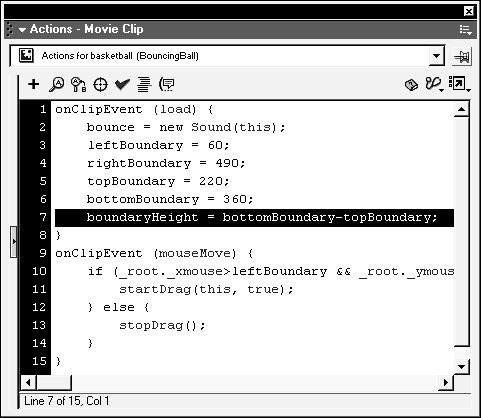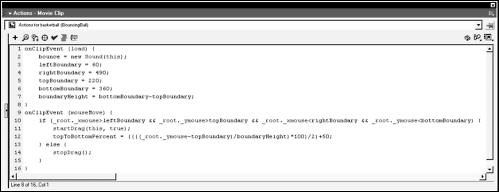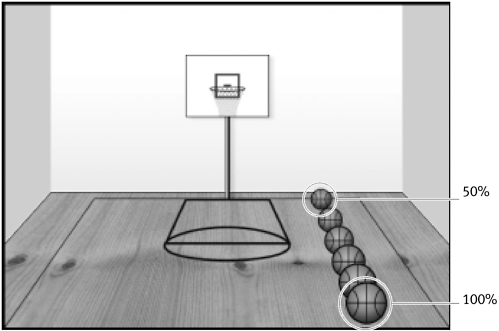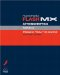# CONTROLLING VOLUME

 Everything we've done to this point has been in preparation for the next several exercises. Although controlling the volume of a movie clip instance with an attached Sound object is pretty straightforward, we plan to take an extremely dynamic approach to the process. You'll remember that in the first exercise in this lesson, we created a Sound object named bounce and associated it with the basketball movie clip instance. This movie clip instance contains a bounce sound that plays when the ball appears to hit the floor. To adjust the volume of this Sound object, you would use the following syntax: ` bounce.setVolume (70); ` This line of script uses the setVolume() method to set the volume of the bounce Sound object to 70 percent. Because this particular Sound object is associated with the basketball movie clip instance, the volume of all sounds on that timeline will be adjusted accordingly. Volume can be set anywhere from 0 (muted) to 100 (100 percent). Because ActionScript is such a dynamic scripting language, we can also use a variable name to set the volume rather than hard-coding it as demonstrated above. Thus, if the value of the variable were to change, so too would the amount of the volume adjustment. Take a look at the following example: ` bounce.setVolume (myVariable); ` This line of script adjusts the volume of the bounce Sound object to the current value of myVariable . As the value of myVariable changes, so does the volume of the bounce Sound object. This dynamic approach is the one we'll be using for this exercise. When you look at our project's background, you'll notice it was designed to provide a sense of depth, with the bottom of the basketball court giving a sense of closeness and the top of the court providing a sense of distance. The court itself is a close visual representation of the boundary we scripted in the previous lesson. With this in mind, our goal is simple: We want to set the volume of the bounce Sound object based on the vertical position of the ball within our boundary. This means that when the ball is at the top of the boundary, the bounce sound will be at 50 percent volume, giving a sense of distance. As the ball moves toward the bottom of the boundary, the bounce sound should get louder (to a maximum of 100 percent), so that the ball sounds like it's getting progressively closer.To achieve this, we need to do a couple of things: First, we want to create a variable and constantly update its value to a number between 50 and 100. We will determine this value by figuring the vertical distance (as a percentage value) between the mouse and the top side of our boundary in relation to the overall vertical size of the boundary. Sound confusing? Let's review the formula for figuring percentages as well as take a look at an example scenario. Here's the formula we'll be using: Determine the overall length of an area. Determine the length of the portion for which the percentage must be figured. Divide the size of the portion by the overall size, then multiply by 100. Using a sample scenario, suppose the mouse has a vertical position of 310. From the previous exercise, we know that our top boundary is 220 and our bottom boundary is 360. With the first part of our formula, we determine the overall length of an area that is, the vertical size of our boundary. You can figure this out by subtracting 220 (the top boundary) from 360 (the bottom boundary). This gives us a value of 140 (360 220). Next, we need to determine the length of the portion for which the percentage must be figured. We get this by subtracting 220 (the top boundary) from 310 (the current position of the mouse). This gives us a value of 90 (310 220), which means that the mouse is currently 90 pixels from the top boundary. Lastly, we divide the size of the portion (90) by the overall vertical size of the boundary (140), then multiply that result by 100. Mathematically, our equation would look like this. (90 / 140) * 100 = x (.6428) * 100 = x x = 64.28 or 64.28%If x were a variable in our movie, we could set the volume of our bounce Sound object to the value of this variable by using the following syntax: ` bounce.setVolume (x) ` Since the value of x is currently 64.28, the volume of the bounce Sound object is set to that value accordingly. However, because the mouse is constantly moving, the value of x is always changing as is the volume of the bounce. We have one more mathematical issue to deal with. Using our current percent-generating equation, we have a percentage range between 0 and 100, with 0 indicating that the mouse is at the top of the boundary and 100 indicating that it's at the bottom. We want a percentage range between 50 and100, where 50 is generated when the mouse is at the top of the boundary and 100 when it's at the bottom. We can easily accomplish this by dividing the percentage value generated (0 to 100) by 2, then adding 50. Take a look at the following examples to see what effect this has on 0-100 values: 50 / 2 = 25 25 + 50 = 75 Using the conversion formula, you can see how a value of 50 (normally midway between 0 and 100) is converted to 75 (midway between 50 and 100). Let's look at one more example: 20 / 2 = 10 10 + 50 = 60 Once again, while a value of 20 is one-fifth the value between 0 and 100, the converted value of 60 is one-fifth the value between 50 and100. At this point, the overall logic we will use to accomplish volume control looks like this: Each time the mouse is moved, If the mouse is within the boundary, Determine the vertical distance (as a percentage between 0 and 100) of the mouse from the top boundary. Divide this value by 2, then add 50. Plug this value into a variable. Use this variable's value to set the volume of the bounce Sound object. It's now time to add this functionality to our movie. Open basketball3.fla in the Lesson16/Assets folder. If you wish, you can simply continue using the file you were working with for the previous exercise. Select the basketball movie clip instance, then open the Actions panel. After the line of script defining the sides of the boundary (which we created in the previous exercise), add the following lines of script: ` boundaryHeight = bottomBoundary topBoundary; `This creates a variable named boundaryHeight and assigns it a value based on what bottomBoundary topBoundary evaluates to. The two lines of script directly above this indicate that bottomBoundary = 360 and topBoundary = 220 . Thus, this line of script written out would look like the following: boundaryHeight = 360 220 or boundaryHeight = 140 This value represents the vertical size of our boundary and will be used to determine percentage values as described previously. TIP Although we could have easily assigned a value of 140 to the boundaryHeight variable directly, using an expression as we've done is much more dynamic. This way, if you ever change the values of topBoundar y or bottomBoundary, the value of boundaryHeight would automatically update accordingly. A well-thought-out script will contain few hard-coded variables; thus, be conscious of where you can use expressions. Place the following line of script after the startDrag (this, true) action: ` topToBottomPercent = ((((_root._ymouse topBoundary) / boundaryHeight) * 100) / 2) + 50; `This line creates the variable topToBottomPercent and assigns it a value based on an expression. This expression is the mathematical representation of the percent formula we discussed earlier. Three dynamic values are needed in order for this expression to be evaluated: _root._ymouse (the vertical position of the mouse), topBoundary (which currently equals 220), and boundaryHeight (which currently equals 140). The multiple parentheses denote the order in which each part of the expression is evaluated. The following demonstrates how this expression is evaluated: _root._ymouse topBoundary is evaluated. The result is divided by boundaryHeight. The result of step 2 is multiplied by 100. The result of step 3 is divided by 2. 50 is added to the result of step 4. There are two unique aspects of the location of this line of script. First, you'll notice it's nested within the mouseMove event handler. This means the expression that determines the value of topTopBottomPercent is evaluated almost every time the mouse is moved and thus the value of the variable is constantly changing based on the current position of the mouse. We say almost, because you'll notice that this line of script is also nested within our if statement that checks to see if the mouse is within the boundary we created. This means that this variable's value is only set/updated when the mouse is within this boundary. Since this variable's value will soon be used to set the volume of the bounce Sound object, you should understand that nesting it within the if statement will prevent changes to the volume of the sound whenever the mouse is outside of the boundary. Place the following line of script after the line we just added (the one that created the topToBottom variable): ` bounce.setVolume(topToBottomPercent); ` This line simply sets the volume of the bounce Sound object, based on the current value of the topToBottomPercent variable. Since the value of this variable is being updated constantly, so too will the volume of the bounce Sound object. Because this line is nested within the mouseMove event handler as well as in the if statement that looks for movement within our boundary, the volume of the bounce Sound object is updated each time the mouse is moved within the boundary. Place the following line of script after the line we just added (the one that set the volume of the bounce Sound object): ` this._xscale = topToBottomPercent; this._yscale = topToBottomPercent; ` These two lines of script add a bonus effect to our project. Using the current value of the topToBottomPercent variable, these lines will adjust the _xscale and _yscale properties of the basketball movie clip instance (this ). Thus, the ball will be scaled in size at the same time the volume of the bounce Sound object is set. In other words, while the volume is being adjusted to provide an auditory sense of the ball's movement, the ball's size is being visually adjusted as well, giving the project a greater sense of reality.Since these lines are nested within the mouseMove event handler as well as in the if statement that looks for movement within our boundary, the size of the ball movie clip instance is updated each time the mouse is moved within the boundary. Choose Control > Test Movie. In the testing environment, move your mouse around the court. As the ball is dragged upward, its size and bounce volume decrease, making it seem that the ball is moving away. As the ball is dragged downward, its size and bounce volume increase, making it seem as if the ball is moving closer. Close the testing environment to return to the authoring environment. Save the current file as basketball4.fla. You may want to keep this file open since we'll continue to build on it as this lesson progresses.Macromedia Flash MX ActionScripting: Advanced Training from the Source
ISBN: 0201770229
EAN: 2147483647
Year: 2002
Pages: 161